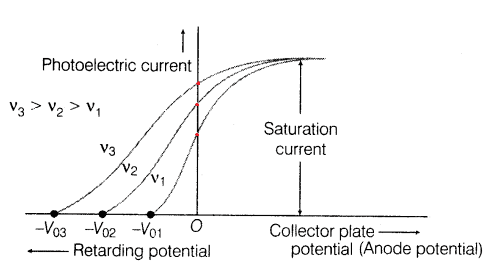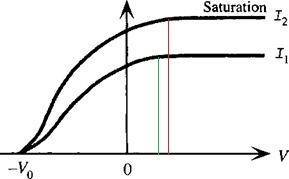# 2 questions about the photo electric effect

• B
• Supitha
In summary, the difference between the red and green lines in the first figure is due to the "work function" of the metal, which determines the likelihood of electrons escaping when exposed to different frequencies of light. The second figure shows two different intensities at the same wavelength, with the "stopping potential" remaining the same due to the same photon energy. However, the more intense light results in more electrons being liberated per second. In all curves, increasing the applied voltage will not significantly affect the number of electrons being pulled, leading to a saturation point.

#### Supitha1. How can explain the difference of these red dots?
2. Red line = Green line ?. How to explain it?

•Kizer
Even when the applied voltage is zero, there is a kind of "virtual" voltage that pulls the electrons back into the metal. This is called the "work function", I believe.
So at low photon frequencies, there is a smaller chance that an electron will end up with enough energy to leave the metal (because total energy = photon energy + random thermal energy). For high photon energies, there is more chance of escaping i.e. more chance that electron's original thermal energy plus photon energy will carry it over the work function threshold.

•Supitha and Kizer
Swamp Thing said:
Even when the applied voltage is zero, there is a kind of "virtual" voltage that pulls the electrons back into the metal. This is called the "work function", I believe.
So at low photon frequencies, there is a smaller chance that an electron will end up with enough energy to leave the metal (because total energy = photon energy + random thermal energy). For high photon energies, there is more chance of escaping i.e. more chance that electron's original thermal energy plus photon energy will carry it over the work function threshold.
Thank you so much.

•Kizer
What's happenning in second one?

Kizer said:
who me?
No. The second pic

I'm guessing here, but the second figure probably shows two different intensities at the same wavelength. The "stopping potential" is the same for both curves because the photon energy is the same, but once you allow some current to flow then the more intense light means more photons per second which means more electrons per second.

Going back to fig. 1, all curves involve the same intensity (same rate of photons per second) so they all saturate at the same number of electrons per second. If you increase the applied voltage to a huge value, you can still pull only those electrons that are liberated by photons, so "pulling harder" won't make much difference -- so the curves saturate at some point.

•Supitha